• 累计撰写 145 篇文章
• 累计创建 37 个标签
• 累计收到 18 条评论

### 目 录CONTENT# 马拉车算法，其实并不难!!!2021-10-12 / 0 评论 / 0 点赞 / 252 阅读 / 2,608 字

## 例子

示例 1：



## 马拉车算法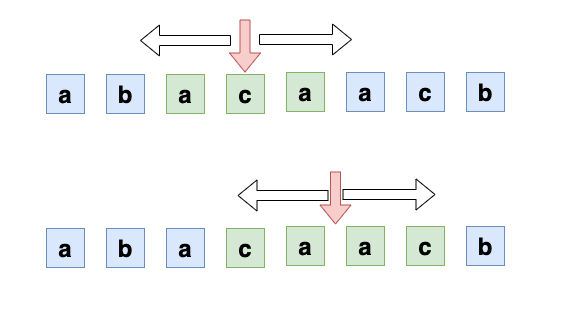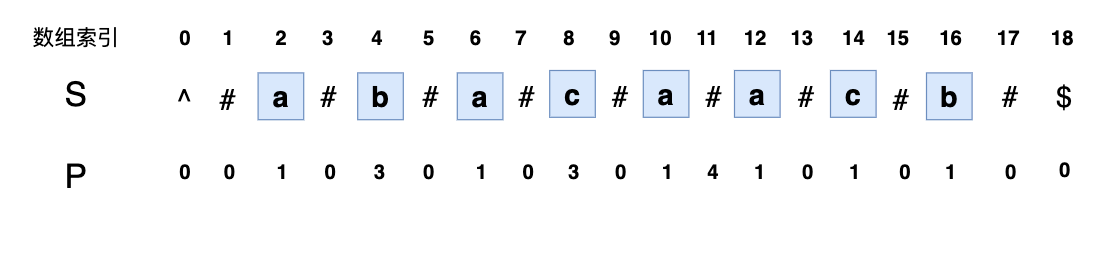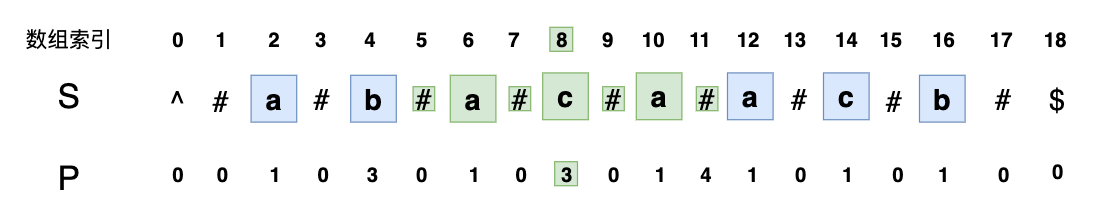P= 4，表示可以往两边分别拓展4个字符，也就是回文串的长度为 4，去掉 # 之后的字符串为caac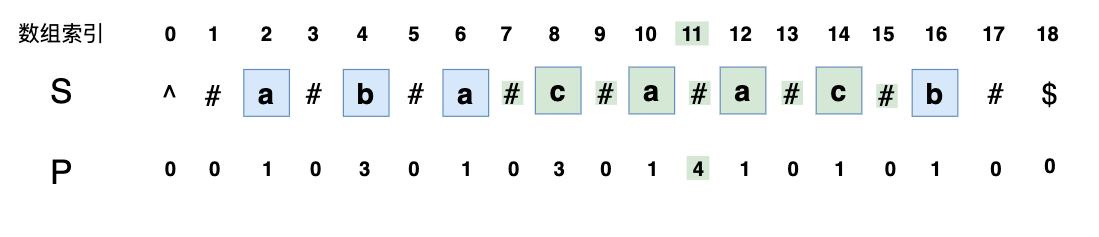P 的下标 index ，减去P[i]（也就是回文串的长度），可以得到回文串开头字符在拓展后的字符串 S 中的下标，除以2，就可以得到在原字符串中的下标了。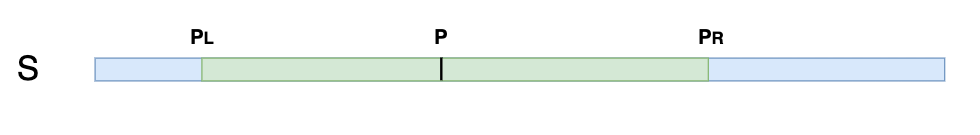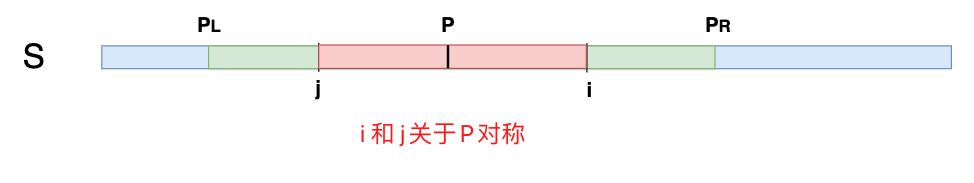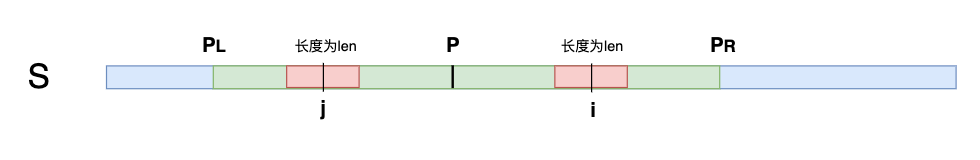• 前一个回文字符串P，是哪一个？
• 有哪些特殊情况？特殊情况怎么处理？

(1) 前一个回文字符串 P，是指的前面计算出来的右边界最靠右的回文串，因为这样它最可能覆盖我们现在要计算的 i 为中心的索引，可以尽量重用之前的结果的对称性。

(2) 特殊情况其实就是当前 i 的最长回文字符串计算不能再利用 P 点的对称，例如：

1. i 的回文串的右边界超出了 P 的右边界 PR: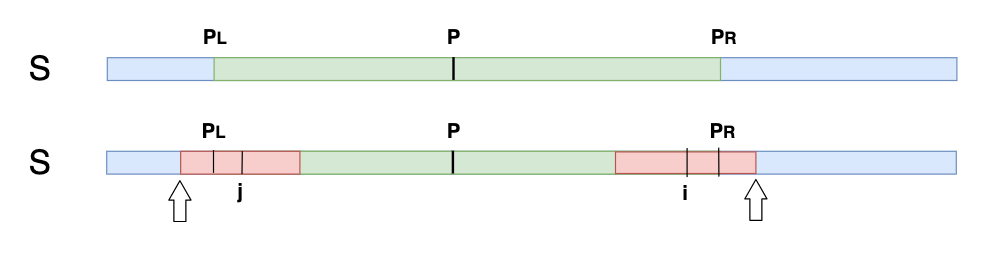1. i 不在 以 P 为中心的回文串里面，只能按照中心拓展法来处理。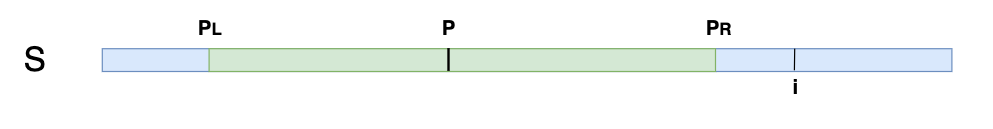// 构造字符串
public String preProcess(String s) {
int n = s.length();
if (n == 0) {
return "^$"; } String ret = "^"; for (int i = 0; i < n; i++) ret = ret + "#" + s.charAt(i); ret = ret + "#$";
return ret;
}

// 马拉车算法
public String longestPalindrome(String str) {
String S = preProcess(str);
int n = S.length();
// 保存回文串的长度
int[] P = new int[n];
// 保存边界最右的回文中心以及右边界
int center = 0, right = 0;
// 从第 1 个字符开始
for (int i = 1; i < n - 1; i++) {
// 找出i关于前面中心的对称
int mirror = 2 * center - i;
if (right > i) {
// i 在右边界的范围内，看看i的对称点的回文串长度，以及i到右边界的长度，取两个较小的那个
// 不能溢出之前的边界，否则就得中心拓展
P[i] = Math.min(right - i, P[mirror]);
} else {
// 超过范围了，中心拓展
P[i] = 0;
}

// 中心拓展
while (S.charAt(i + 1 + P[i]) == S.charAt(i - 1 - P[i])) {
P[i]++;
}

// 看看新的索引是不是比之前保存的最右边界的回文串还要靠右
if (i + P[i] > right) {
// 更新中心
center = i;
// 更新右边界
right = i + P[i];
}

}

// 通过回文长度数组找出最长的回文串
int maxLen = 0;
int centerIndex = 0;
for (int i = 1; i < n - 1; i++) {
if (P[i] > maxLen) {
maxLen = P[i];
centerIndex = i;
}
}
int start = (centerIndex - maxLen) / 2;
return str.substring(start, start + maxLen);
}


【作者简介】

2020年我写了什么？

0